Home > CC2MN > Chapter cc29 > Lesson cc29.2.2 > Problem9-70

9-70.
1. Evaluate the inequality x + 4 < 2x − 3 for the following values of x. Decide if the given value of x makes the statement true or false. Show all of your work. Homework Help ✎

1. x = 4

2. x = −2

3. x = 7

4. x = 9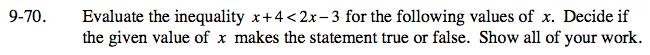Substitute each value for x in the inequality given above.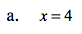4 + 4 < 2(4) − 3

Simplifying, you get:
8 < 8 − 3
8 < 5
Is 8 less than 5?

False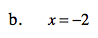−2 + 4 < 2(−2) − 3

Simplifying, you get:
2 < −4 − 3
2 < −7
Is 2 less than -7?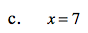See parts (a) and (b).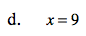See parts (a) and (b).